Select Page

# (with illustrations)

written by Stanley Udegbunam || Jan 15th, 2021## What is the direction of Static Friction?

Static Friction acts opposite the direction of the net applied force.

This friction type exists between two surfaces at rest relative to each other.

As a result of this, the direction of static friction cannot be directly visualized like kinetic friction.

Nevertheless, friction force in whatever form, is a resistive force and will always act opposite the direction of motion.

In a hurry?

Here’s a quick infographic summary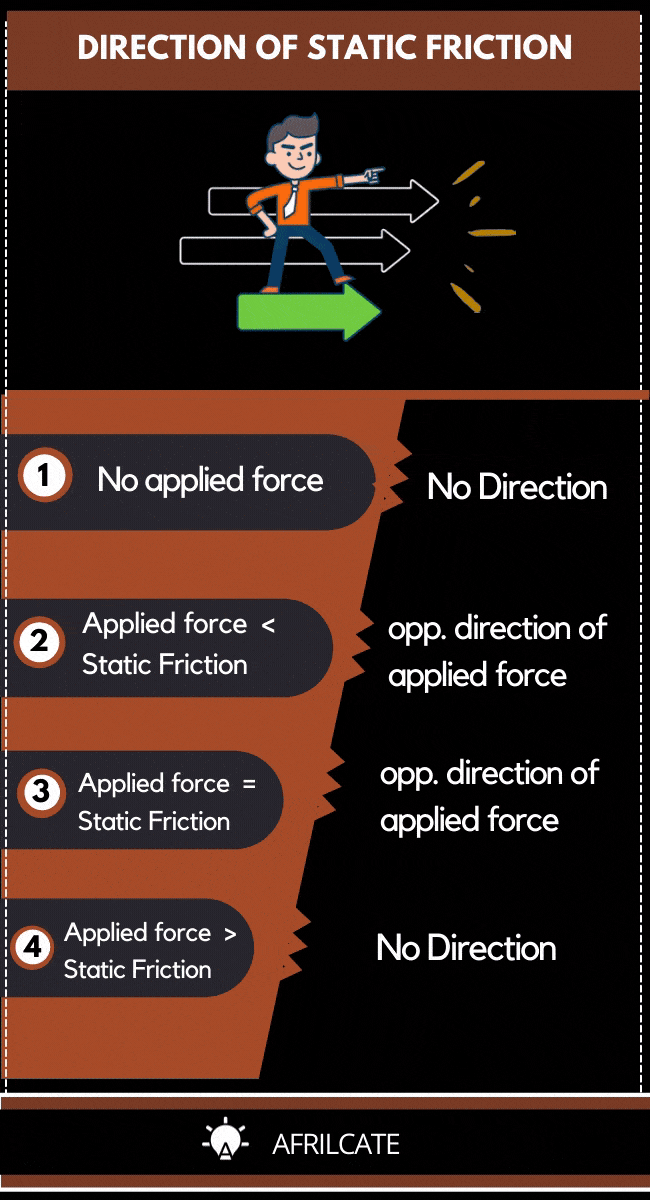Let’s dive deep by analyzing different case scenarios.

### CASE 1

Consider a wooden box of weight 200N sitting undisturbed on the floor with a coefficient of static friction, 0.3N.The maximum value of static friction = Coefficient of Friction × Normal force

Fs = µs × N

Normal force = weight of the object

Maximum value of static friction = 0.3 x 200 = 60N

The maximum value of static friction is also known as limiting friction force.

Let’s draw the free body diagram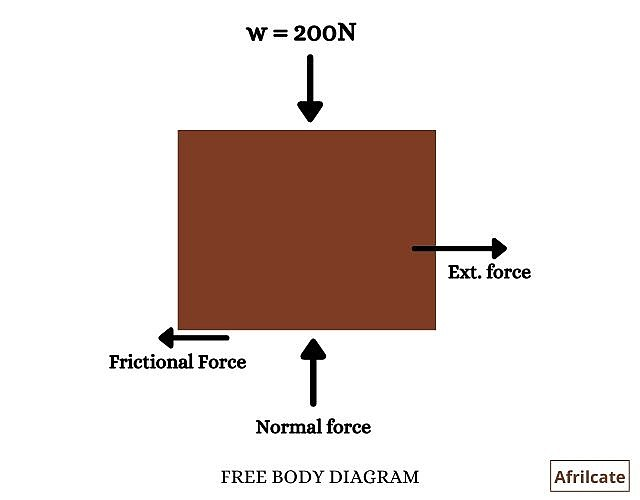### Q1. What is the direction of static friction when there’s no applied force?

Since no force is applied, static friction force will not exist or have a zero value, a state called static friction zero.

Static friction is a vector meaning it possesses both magnitude and direction.

It is direction is dependent on the direction of the applied force.

When there’s no applied force, static friction has no direction.

At static friction zero, it has no magnitude and direction.

### CASE 2

Let’s consider a block of 500N on a wooden basement with a coefficient of friction of 0.6

Maximum value of static friction = 0.6 x 500 = 60N

A lady tries to move the block over a distance by applying a force of 40N which is less than the maximum value of static friction.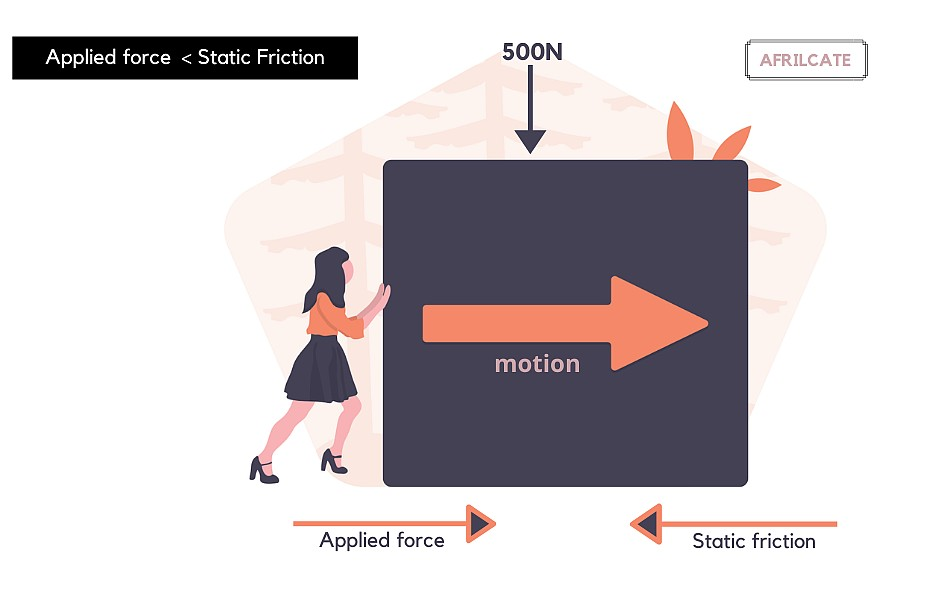### Q2. What will be the direction of static friction when the applied force is less than the maximum value of static friction?

The direction of static friction will be opposite the direction of the applied force.

Since the applied force is less than the maximum value of static friction, the object won’t move.

For motion to occur, the applied force must be greater than the limiting static friction.

FREE BODY DIAGRAM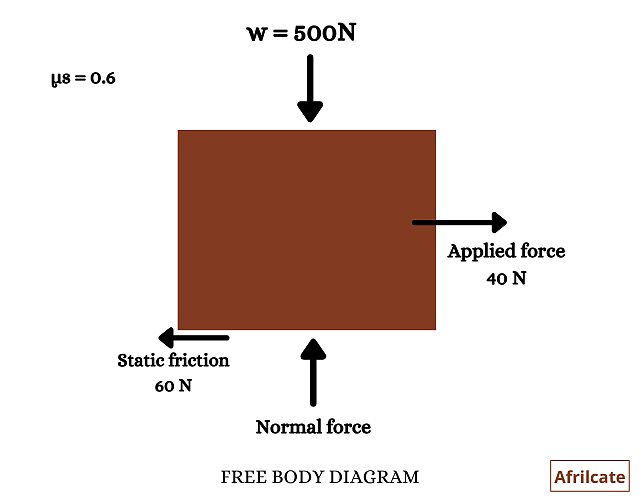### CASE 3

Using the same scenario above, let’s assume the lady applies a force of 60N which is the same as the maximum static friction value.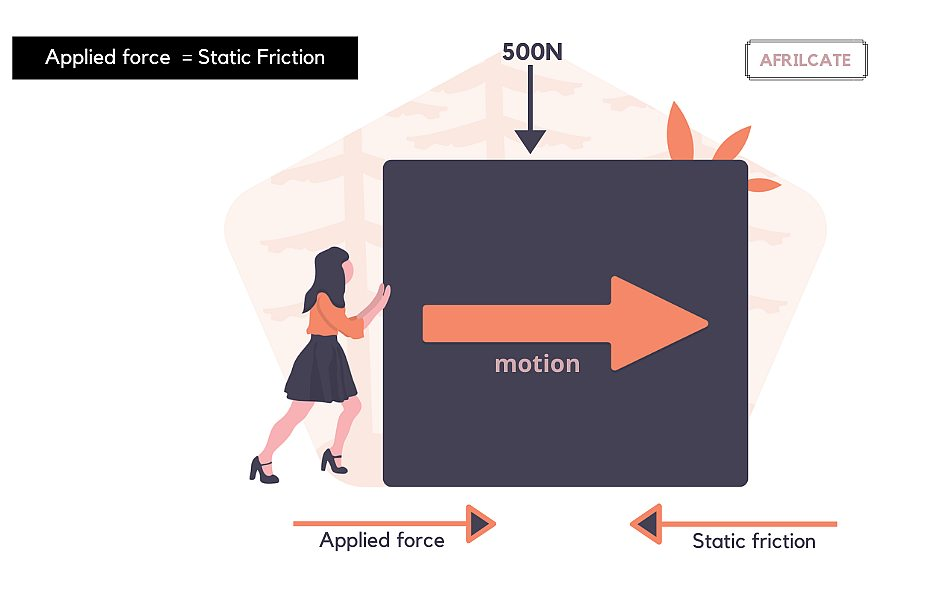### Q3. What will be the direction of static friction if the applied force is the same as the maximum value of static friction?

The direction of static friction will still be opposite that of the applied force.

At this point, the force applied is equal to the limiting force which is the transition phase of the stationary position.

This transition phase is known as the threshold of motion.

Any additional force applied regardless of its magnitude will cause the object to move.

FREE BODY DIAGRAM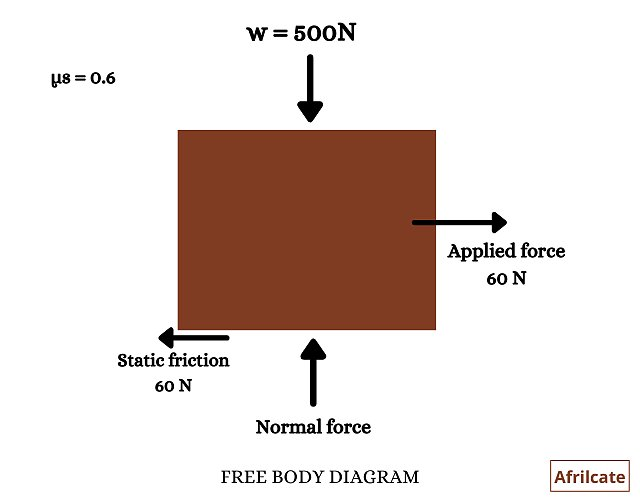### CASE 4

I guess you know where we are headed by now.

Let’s assume the lady applies a force of 70N which happens to be greater than the maximum value of static friction.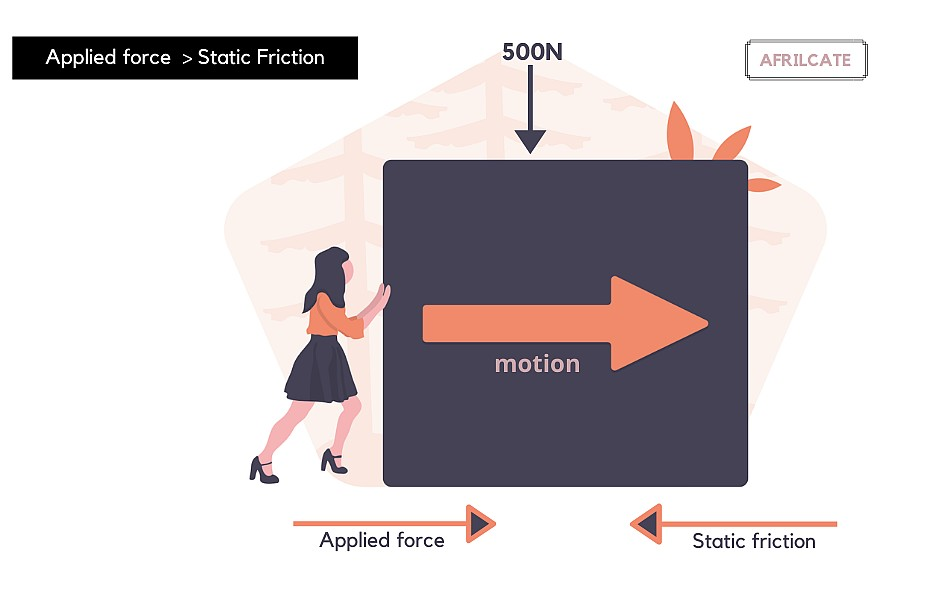### Q4. What will be the direction of static friction if the applied force is greater than the maximum value of static friction?

Since the applied force is greater than the limiting friction, the object will move in the direction of the force and static friction ceases to exist.

Once the threshold of motion is exceeded by the applied force, the opposing force changes from static friction to kinetic friction.

Therefore, the static friction has no direction because the state of the object has been changed.

FREE BODY DIAGRAM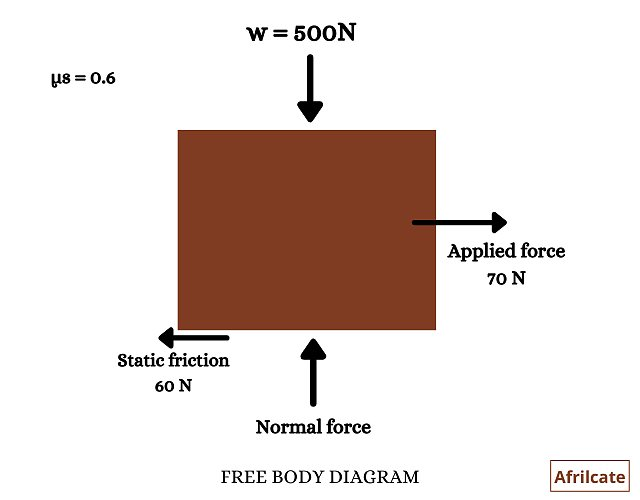From the 4-case scenario, you can see that we can only talk about the direction of static friction when the object is in a stationary position.

And it actually makes sense because static friction acts between surfaces at rest relative to each other.

Anything beyond that means exceeding the scope of its definition.

Let’s have a quick question summary:

1. What is the direction of static friction when there’s no applied force?

Since no force is applied, static friction force will not exist or have a zero value, a state called static friction zero.

Since the static friction has no value, it, therefore, possesses no direction.

1. What is the direction of static friction when the applied force is less than the maximum value of static friction:

The direction of static friction will be opposite the direction of the applied force.

1. What will be the direction of static friction if the applied force is the same as the maximum value of static friction?

The direction of static friction will still be opposite that of the applied force.

1. What will be the direction of static friction if the applied force is greater than the maximum value of static friction?

The force applied will cause motion, static friction will cease to exist and possess no direction.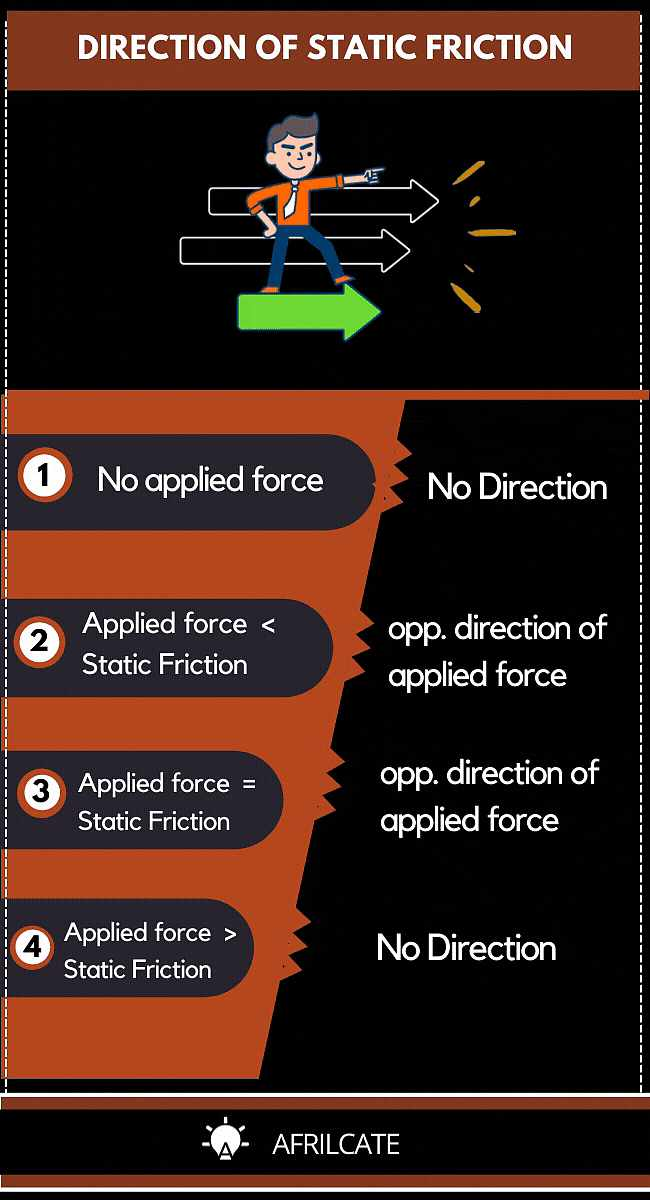Still curious about static friction? see our guides:

1. STATIC FRICTION : The Complete Guide